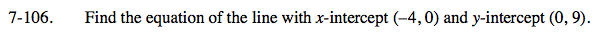Home > CAAC > Chapter 7 > Lesson 7.3.3 > Problem7-106

7-106.

Find the equation of the line with x-intercept (−4, 0) and y-intercept (0, 9). Homework Help ✎y = mx + b

Substitute the y-coordinate of the y-intercept for b in the equation.

Make a slope triangle to find the slope of the line, then substitute that value for m to finish the equation.

$y=\frac{9}{4}x+9$